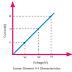Hey, in this article we are going to discuss about Linear Elements and Non-Linear Elements. According to the nature of flow of current through elements when voltage across them, electrical or electronic elements are divided into two types - 1. Linear Element 2. Non-Linear Element. Here we will know the examples, properties or characteristics, and uses or applications.

## What is Linear Element?

Linear elements are those through which the flow of current changes linearly with the changing of the applied voltage across them. This can be understood by observing the voltage-current graph of a linear element. The voltage-current graph of the linear element is always a straight line. This straight line shows that increase in current is linear to the increasing in voltage.

## Linear Element Examples

A resistor is the best example of Linear Element. Capacitor and Inductor are also examples of Linear Element.

## Linear Element Properties

1. Linear Elements obeys ohm's law.
2. The voltage-current relation is linear.
3. The voltage-current graph is straight.
4. Linear Elements obeys the superposition theorem.
5. The current flow through the Linear elements will be uniform.

## Applications and Uses of Linear Element

The main function of linear elements to oppose the flow of current, or store the electric energy, or convert the electrical energy into other form. So, linear elements are used for current limiting, filtering, energy storing, and energy conversion applications.

## What is Non-Linear Element?

Non-Linear Elements are those through which, the flowing current does not change linearly with the changing of the applied voltage across them. This can be understood better by observing the voltage-current graph of the non-linear element. The voltage-current graph of the non-linear element not a straight line like the linear element, most of the cases it is exponential. This exponential line indicates that an increase in current does not change linearly or uniformly with the increase of applied voltage.

## Non-Linear Element Examples

PN junction Diode is the best example of non-linear element. Generally, all the semiconductor devices such as BJT, FET can be called examples of Non-Linear Elements.

## Non-Linear Element Properties

1. Generally, non-linear elements do not obey Ohm's Law.
2. Voltage current relation is non-linear.
3. Voltage current graph is not a straight line, in most of the cases, it is a straight line.
4. Non-Linear elements do not obey ohm's law.
5. The current flow through the non-linear elements may not be uniform.

## Applications and Uses of Non-Linear Element

The main function of a non-linear element is to control the flow of current through it. That's why you can see most of the linear elements are current-controlled. Anyway, non-linear elements are used as a current source, current control, rectification, frequency control applications.

## Difference between Linear and Non-Linear Elements

1. The current flow through the linear element is uniform but the current flow through the non-linear element is not uniform.

2. Linear elements obeys ohm's law but non-linear elements do not obey ohm's law.

3. The main function of a linear element is to oppose the current flow or energy storing or energy conversion whereas the main function of a non-linear element is to control the flow of current through with different conditions applied to it.

4. The voltage-current relation is linear for the linear element whereas the voltage-current relation is not linear for the non-linear element.

Linear and Non Linear Elements Examples, Properties, UseReviewed by Author on 8/01/2021 Rating: 5